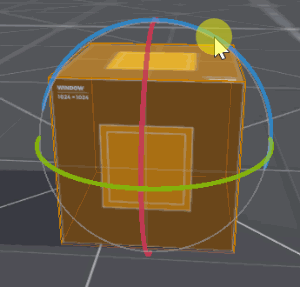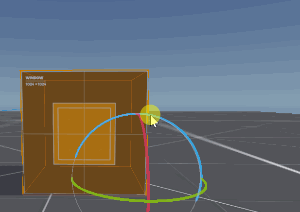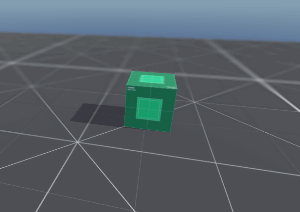# Rolling Cube

## Problem

You want to make a rolling cube in 3D.

## Solution

Rolling a cube is trickier than it seems. You can’t just rotate the cube around its center:Instead, the cube needs to be rotated around its bottom edge.Here’s the tricky part: which bottom edge? It depends on which direction the cube is rolling.

In preparing this recipe, I experimented with a few different solutions to this problem:

• Pure math - calculating and applying rotation transforms
• AnimationPlayer - using animations to key the rotations and offsets
• Helper nodes - using Spatial(s) as rotation helpers

They all worked fine, but I found the last option the most flexible and easiest to adapt, so that’s what we’ll do here.

### Node setup

``````Cube:  KinematicBody
Pivot:  Spatial
Mesh:  MeshInstance
Collision:  CollisionShape
Tween:  Tween
``````

By default, everything will be centered at `(0, 0, 0)` so the first thing we’re going to do is offset everything so that the bottom center of the cube is the `KinematicBody`’s position.

To do this, move the mesh and collision nodes up to `(0, 1, 0)`, leaving the rest where they are. Now when you select the root node, its position will be the bottom of the cube:Now when you want to roll the cube, you’ll need to move the `Pivot` one unit in the direction you want to move. Since the mesh is attached, move it the opposite amount. For example, to roll to the right (+X), you’ll end up with this:Now the pivot node is at the correct edge and rotating it will also rotate the mesh.

### Movement script

The movement is broken in to 3 steps:

#### Step 1

Here we apply the two offsets shown above: shift the `Pivot` in the direction of movement, and shift the `Mesh` in the opposite direction.

#### Step 2

In this step we animate the rotation. We find the axis of rotation using the cross product of the direction and the down vector. Then we use the `Tween` to animate rotating the pivot’s basis. We use `yield()` to wait for the animation to finish.

#### Step 3

Finally, we need to reset everything so that it’s ready to happen again. In the end, we want to have the cube moved 2 units in the chosen direction (for a cube of size 2) and the pivot and mesh back at their original positions.

``````extends KinematicBody

export var speed = 4.0

onready var pivot = \$Pivot
onready var mesh = \$Pivot/MeshInstance
onready var tween = \$Tween

func _physics_process(_delta):
var forward = Vector3.FORWARD
if Input.is_action_pressed("up"):
roll(forward)
if Input.is_action_pressed("down"):
roll(-forward)
if Input.is_action_pressed("right"):
roll(forward.cross(Vector3.UP))
if Input.is_action_pressed("left"):
roll(-forward.cross(Vector3.UP))

func roll(dir):
# Do nothing if we're currently rolling.
if tween.is_active():
return

## Step 1: Offset the pivot
pivot.translate(dir)
mesh.translate(-dir)

## Step 2: Animate the rotation
var axis = dir.cross(Vector3.DOWN)
tween.interpolate_property(pivot, "transform:basis",
null, pivot.transform.basis.rotated(axis, PI/2),
tween.start()
yield(tween, "tween_all_completed")

## Step3: Finalize movement and reverse the offset
transform.origin += dir * 2
pivot.transform = Transform.IDENTITY
mesh.transform.origin = Vector3(0, 1, 0)
``````

Try it, and you’ll notice there’s one problem:The cube is “squishing” because of the basis interpolation, which is going from 0 to 90 degrees. Since the basis also represents scale, this causes some deformation.

We could solve this in 2 ways. First, we could break up the rotation into two separate tweens, each doing 45 degrees. However, I find this tricky when we want to play around with the different tween transitions.

The other way is to ensure the scale remains constant. We can do this by connecting the `Tween`’s `tween_step` signal, which fires every frame during the tween, and normalizing the scale. The `orthonormalized()` method does this:

``````func _on_Tween_tween_step(_object, _key, _elapsed, _value):
pivot.transform = pivot.transform.orthonormalized()
``````

If your cube’s texture isn’t symmetrical, you may notice that it’s resetting after every roll. To preserve the rotation of the mesh, add the following:

In Step 1

Change `mesh.translate(-dir)` to `mesh.global_translate(-dir)`.

In Step 3

Add two lines to keep the mesh rotation after reset:

``````## Step3: Finalize movement and reverse the offset
transform.origin += dir * 2
var b = mesh.global_transform.basis  ## Save the rotation
pivot.transform = Transform.IDENTITY
mesh.transform.origin = Vector3(0, 1, 0)
mesh.global_transform.basis = b  ## Apply the rotation
``````

### Checking for collisions

If you plan to have obstacles in your game, you can check for collisions before moving (similar to any other grid-based movement scheme). Add a raycast check before Step 1 of the move:

``````# Cast a ray before moving to check for obstacles
var space = get_world().direct_space_state
var collision = space.intersect_ray(mesh.global_transform.origin,
mesh.global_transform.origin + dir * 2.5, [self])
if collision:
return
``````